Fall 2004

# Mathematics Math21b Fall 2004

## Linear Algebra and Differential Equations

Office: SciCtr 434
Email: knill@math.harvard.edu

# Surfaces and Linear Algebra

While the topic of parametrized or implicit surfaces belongs to a multivariable calculus course, linear algebra plays an important role in studing surfaces too. While surfaces are defined by functions which are nonlinear in general, one can look at the linear space of functions which define them. We can for example look at the space of all smoothly parametrized surfaces in three dimensional space parametrized by two parameters u,v and assume that the functions are periodic in u and v. This is a linear space X. We can add and scale functions in this linear space. For example, the functions

 ``` F(u,v) = (sin(u) cos(v), cos(u) sin(v),cos(v) ) G(u,v) = ((2+sin(v)) cos(v), (2+sin(v)) sin(u),cos(v) ) ```

define the two well known surfaces sphere and torus. We can treat F and G as vectors and add and subtract them. What do we get if we add a sphere to a torus? The middle picture shows (F+G)/2. If we scale a function F, this means we also scale the surface.

 F (F+G)/2 G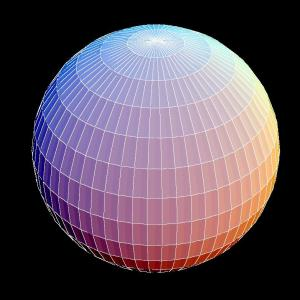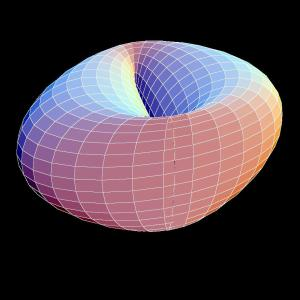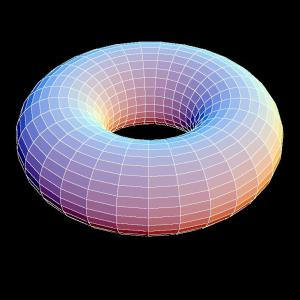Lets look at the line segement in the space X which connects the two "vectors" F and G. What is the use of this? There are many mathematical reasons why one wants to do this - but first of all, it is just beautiful, isn't it?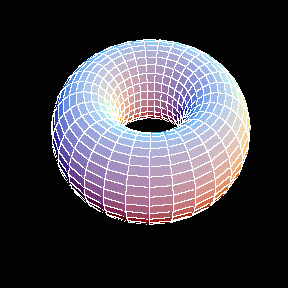The following exposition appeared in the New York Times Magazine on Sunday, December 5, 2005. We tried the same Sunday morning to reproduce the pictures using Mathematica. The source code can be found below. Citation NYT: "It's hard to imagine that these plaster forms, so starkly beautiful, were originally used to teach advanced students trigonometry. Called stereometric models, they were manufactured in turn-of-the-century Germany to help scholars grasp complex mathematical formulas. Last year, the Japanese photographer Hiroshi Sugimoto shot each object, the tallest of which is less than a foot high, from below at close range so that they appear monumental. His series of photographs, 'Mathematical Forms', reimagine these scientific models as things of wonder. They embody Sugimoto's belief that art is possible even without artistic intention." Hiroshi Sugimoto is a Japanese born American Photographer. His commitment to taking photographs that combine the conceptual, the sensual and the technical has made him a rising contemporary art star.

 Photo of Hiroshi Sugimoto Mathematica picture Figure capture New York Times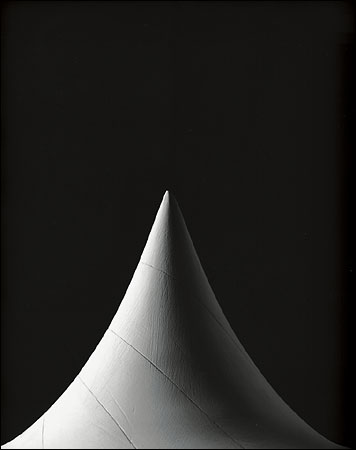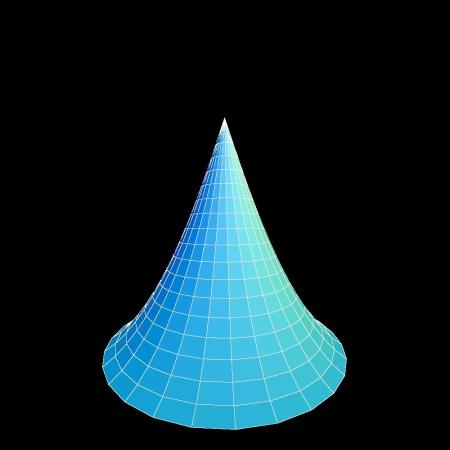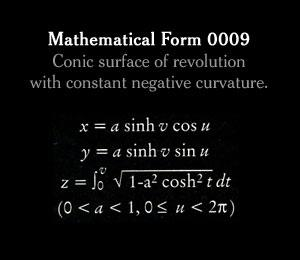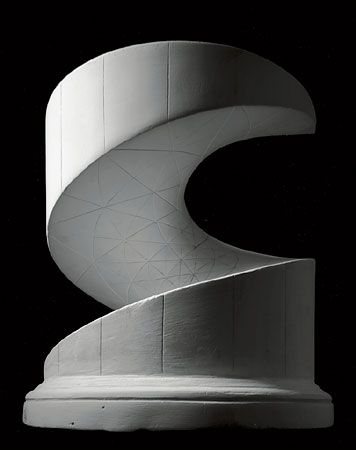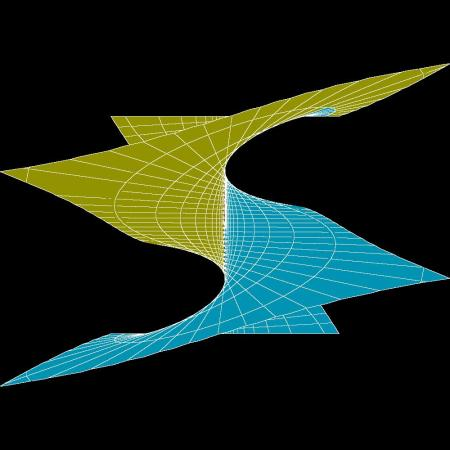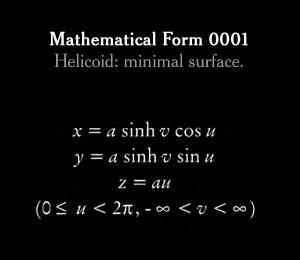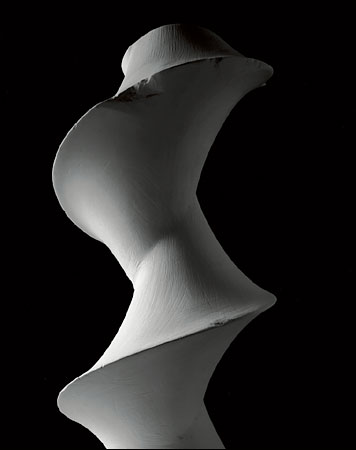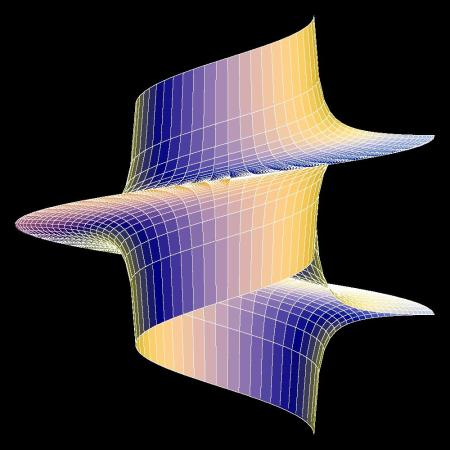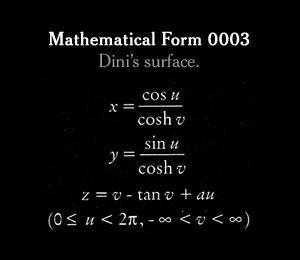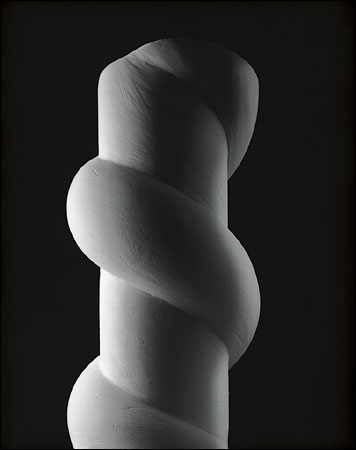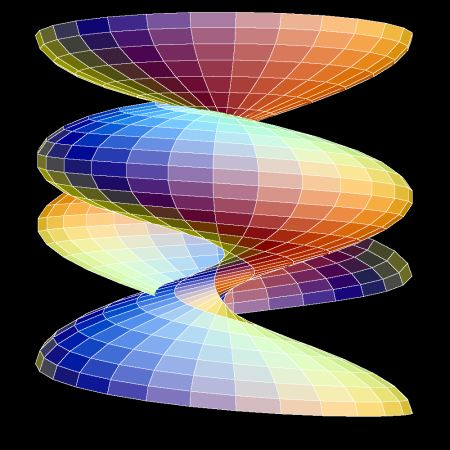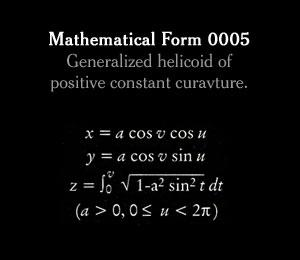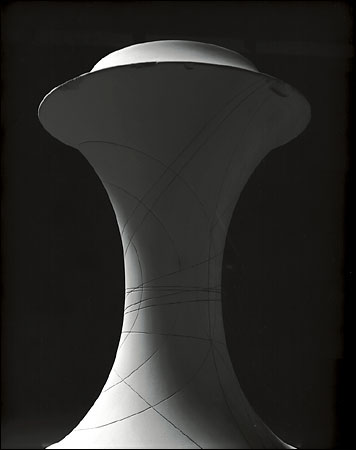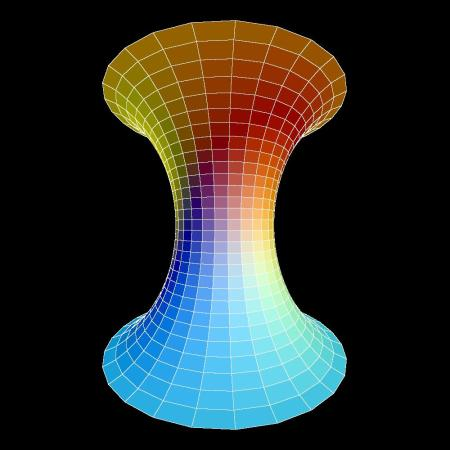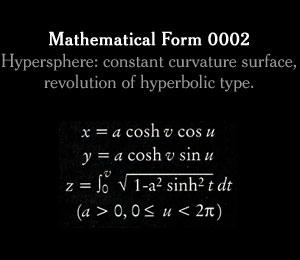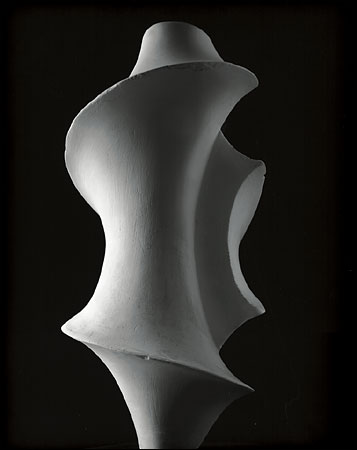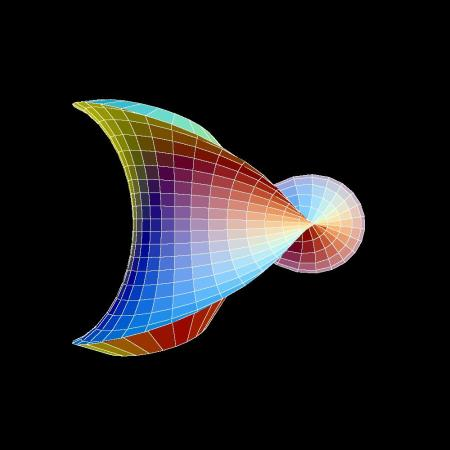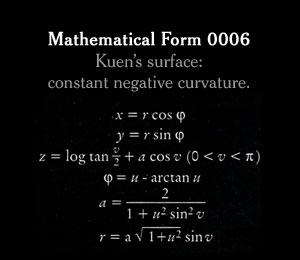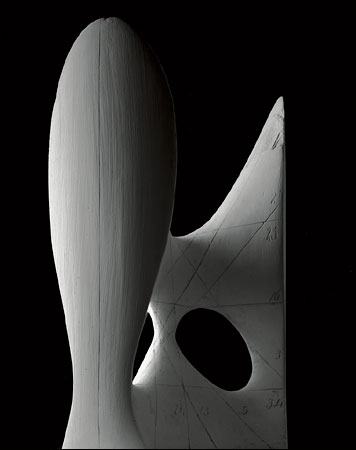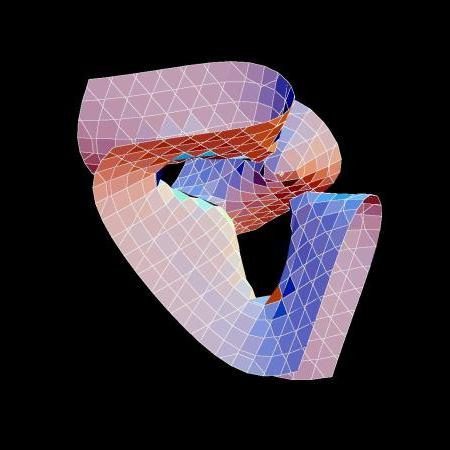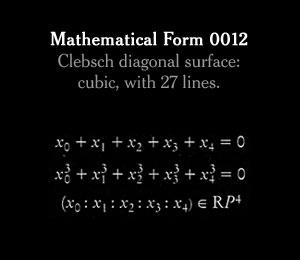## Mathematica Source code

 ```(* Rebuilding the surfaces in Mathematica which appeared in the *) (* New York Times Magazine (Photos of the American Artist *) (* Hiroshi Sugimoto, December 5, 2004 *) (* Oliver Knill, December 5, 2004, for Math21b, Harvard Fall 2004 *) F1[f_,a_,b_,c_,d_,n_]:=ParametricPlot3D[f[u,v],{u,a,b},{v,c,d}, Boxed->False,Axes->False,PlotPoints->n,AspectRatio->1, ViewPoint->{0,1,0},ViewVertical -> {0,0,-1}] f1[u_,v_]:={Sinh[v] Cos[u],Sinh[v] Sin[u],NIntegrate[Sqrt[1-Cosh[t]^2/4],{t,0,v}]} S1=F1[f1,0,2Pi,0,ArcCosh,30] f2[u_,v_]:={Sinh[v] Cos[u],Sinh[v] Sin[u],u} S2=F1[f2,0,2Pi,-10,10,50] f3[u_,v_]:={Cos[u]/Cosh[v],Sin[u]/Cosh[v],v-Tan[v]+3 u} S3=F1[f3,0,2Pi,-1.5,1.5,50] f4[u_,v_]:={Cos[u] Cos[v]*4/5,Sin[u] Cos[v]*4/5, NIntegrate[Sqrt[1-Sin[t]^2*u/(2Pi)],{t,0,v}]} S4=F1[f4,0,2Pi,0,2Pi,30] f5[u_,v_]:={Cosh[v] Cos[u],Cosh[v] Sin[u],NIntegrate[Sqrt[1-Sinh[t]^2/4],{t,0,v}]} S5=F1[f5,0,2Pi,-ArcSinh,ArcSinh,30] f6[u_,v_]:={2 Sqrt[1+u^2] Sin[v] Cos[u-ArcTan[u]]/(1+u^2 Sin[v]^2), 2 Sqrt[1+u^2] Sin[v] Sin[u-ArcTan[u]]/(1+u^2 Sin[v]^2), Log[Tan[v/2]]+2 Cos[v]/(1+u^2 Sin[v]^2)}; S6=F1[f6,0,6,0,4,30] Get["Graphics`ContourPlot3D`"]; eqn = 81 (x^3 + y^3 + z^3) - 189 (x^2y + x^2z + y^2x + y^2z + z^2x + z^2y) + 54x y z + 126 (x y + x z + y z) - 9 1(x^2+y^2+z^2) - 9 (x+y+z)-2; (* eqn from http://www-sop.inria.fr/galaad/exposition/ArtGallery/clebsh.html *) S7=ContourPlot3D[eqn,{x,-1,1},{y,-1,1},{z,-1,1}, Background->GrayLevel[0.0], PlotPoints->5,Boxed->False,Axes->False] ```